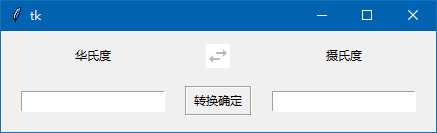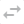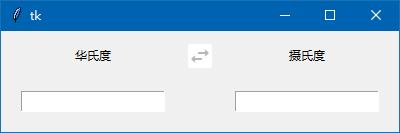# 2种方法做华氏度和摄氏度的转换（tkinter）

### 不用变量的来做温度转换程序界面上一共6个组件。从左到右，从上到下分别是：

标签1，la1，默认text：华氏度

按钮1，but1，image为：标签2，la2，默认textg：摄氏度

文本框1，en1

按钮2，but2，text=” 转换确定 “

文本框2，en2

```from tkinter import *

def zf(): # 2个标签文本互换
str=la1['text']
la1['text']=la2['text']
la2['text']=str

def c2f(): # 根据标签的不同，选择不同的公式来计算结果
a=float(en1.get()) # 获取文本框1的数据

if la1['text']=='华氏度':
b= (a-32)/1.8  # 华氏度=(摄氏度-32)/1.8
else:
b= a*1.8+32    # 摄氏度=华氏度*1.8+32

en2.delete(0,END)  # 先清空文本框2
en2.insert(END,b)  # 再插入计算的结果

root=Tk()
la1=Label(root,text='华氏度')

img1=PhotoImage(file='f2.png')
but1=Button(root,image=img1,relief='flat',cursor='hand2',command=zf) # 绑定标签文本互换

la2=Label(root,text='摄氏度')

en1=Entry(root)

but2=Button(root,text=" 转换确定 ",relief='groove',cursor='hand2',command=c2f) # 绑定计算函数
but2.grid(row=1,column=1)

en2=Entry(root)

root.mainloop()```

tkinter新手，请手工输入代码后，运行后，再跟我的代码对比一下，应该可以很好地巩固前面学的知识。上面的代码都有注释，如还有不清楚的，可以留言给我。我的网站是：wb98.com

```def c2f(): # 根据标签的不同，选择不同的公式来计算结果
a=float(en1.get()) # 获取文本框1的数据（浮点数）```

```def c2f(): # 根据标签的不同，选择不同的公式来计算结果
try:
a=float(en1.get()) # 获取文本框1的数据（浮点数）
except:
en2.delete(0,END) # 清空文本框
en2.insert(END,'输入数据为空或错误')  # 输入为空或错误给予提示
return  # 返回```

### 用变量的追踪方法来做温度转换程序

```from tkinter import *

def zf(): # 2个标签文本互换
str=la1['text']
la1['text']=la2['text']
la2['text']=str

def c2f(*args): # 根据标签的不同，选择不同的公式来计算结果
try:
a=float(en1.get()) # 获取文本框1的数据（浮点数）
except:
en2.delete(0,END) # 清空文本框
en2.insert(END,'输入数据为空或有错误')  # 输入为空或错误给予提示
return  # 返回

if la1['text']=='华氏度':
b= (a-32)/1.8  # 华氏度=(摄氏度-32)/1.8
else:
b= a*1.8+32    # 摄氏度=华氏度*1.8+32

en2.delete(0,END)  # 先清空文本框2
en2.insert(END,b)  # 再插入计算的结果

root=Tk()
la1=Label(root,text='华氏度')

img1=PhotoImage(file='f2.png')
but1=Button(root,image=img1,relief='flat',cursor='hand2',command=zf) # 绑定标签文本互换

la2=Label(root,text='摄氏度')

var=StringVar()  # 文本框1的文本变量
en1=Entry(root,textvariable=var)  # 文本框1文本变量绑定var

var.trace('w',c2f)  # 变量追踪，w模式，绑定c2f函数，注意，函数有参数。

en2=Entry(root)

root.mainloop()```

1. 去除了but2创建代码。

2.  c2f()函数加上了参数，为：c2f(*args)

3.  文本框1创建代码改成：

var=StringVar()  # 文本框1的文本变量

en1=Entry(root,textvariable=var)  # 文本框1文本变量绑定var<< 上一篇 下一篇 >>